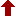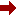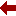#### SLIM21

 Sort Order Chronological Author Title ItemType,Author ItemType,Title Display Format Tabular Publication Bibliography Items / Page 5 10 25

Click the serial number on the left to view the details of the item.
#  AuthorTitleAccn#YearItem Type Claims
11 Wazwaz, Abdul-Majid Partial Differential Equations and Solitary Waves Theory I07354 2009 eBook
12 Derezinski, Jan Large Coulomb Systems I06645 2006 eBook
13 Rodrigues, Waldyr A The Many Faces of Maxwell, Dirac and Einstein Equations I06614 2007 eBook
14 Economou, Eleftherios N Green's Functions in Quantum Physics I06529 2006 eBook
15 Lieb, Elliott H The Stability of Matter: From Atoms to Stars I06244 2005 eBook
16 Gerdjikov, Vladimir Integrable Hamiltonian Hierarchies I06124 2008 eBook
17 Fe??kan, Michal Bifurcation and Chaos in Discontinuous and Continuous Systems I06061 2011 eBook
18 Asch, Joachim Mathematical Physics of Quantum Mechanics I05937 2006 eBook
19 Hassani, Sadri Mathematical Methods I05381 2009 eBook
20 Bohm, Arno Dirac Kets, Gamow Vectors and Gel???fand Triplets I05364 1989 eBook
 (page:2 / 8) [#73] First Page Previous Page Next Page Last Page

11.TitlePartial Differential Equations and Solitary Waves Theory
Author(s)Wazwaz, Abdul-Majid
PublicationBerlin, Heidelberg, Springer Berlin Heidelberg, 2009.
Description700 p. 14 illus : online resource
Abstract Note"Partial Differential Equations and Solitary Waves Theory" is a self-contained book divided into two parts: Part I is a coherent survey bringing together newly developed methods for solving PDEs. While some traditional techniques are presented, this part does not require thorough understanding of abstract theories or compact concepts. Well-selected worked examples and exercises shall guide the reader through the text. Part II provides an extensive exposition of the solitary waves theory. This part handles nonlinear evolution equations by methods such as Hirota???s bilinear method or the tanh-coth method. A self-contained treatment is presented to discuss complete integrability of a wide class of nonlinear equations. This part presents in an accessible manner a systematic presentation of solitons, multi-soliton solutions, kinks, peakons, cuspons, and compactons. While the whole book can be used as a text for advanced undergraduate and graduate students in applied mathematics, physics and engineering, Part II will be most useful for graduate students and researchers in mathematics, engineering, and other related fields. Dr. Abdul-Majid Wazwaz is a Professor of Mathematics at Saint Xavier University, Chicago, Illinois, USA
ISBN,Price9783642002519
Keyword(s)1. ANALYSIS 2. Analysis (Mathematics) 3. APPLIED MATHEMATICS 4. EBOOK 5. EBOOK - SPRINGER 6. ENGINEERING 7. ENGINEERING MATHEMATICS 8. Engineering, general 9. MATHEMATICAL ANALYSIS 10. Mathematical and Computational Engineering
Item TypeeBook
Circulation Data
 Accession# Call# Status Issued To Return Due On Physical Location I07354 On Shelf

12.TitleLarge Coulomb Systems : Lecture Notes on Mathematical Aspects of QED
Author(s)Derezinski, Jan;Siedentop, Heinz
PublicationBerlin, Heidelberg, Springer Berlin Heidelberg, 2006.
DescriptionX, 326 p : online resource
Abstract NoteA mathematically consistent formulation of relativistic quantum electrodynamics (QED) has still to be found. Nevertheless, there are several simplified effective models that successfully describe many body quantum systems and the interaction of radiation with matter. Large Coulomb Systems explores a selection of mathematical topics inspired by QED. It comprises selected, expanded and edited lectures given by international experts at a topical summer school and is intended as a high-level introduction for graduate students, postdocs and nonspecialists from related fields
ISBN,Price9783540325796
Keyword(s)1. ANALYSIS 2. Analysis (Mathematics) 3. EBOOK 4. EBOOK - SPRINGER 5. Elementary particles (Physics) 6. Elementary Particles, Quantum Field Theory 7. MATHEMATICAL ANALYSIS 8. Mathematical Methods in Physics 9. PHYSICS 10. QUANTUM FIELD THEORY
Item TypeeBook
Circulation Data
 Accession# Call# Status Issued To Return Due On Physical Location I06645 On Shelf

13.TitleThe Many Faces of Maxwell, Dirac and Einstein Equations : A Clifford Bundle Approach
Author(s)Rodrigues, Waldyr A;Oliveira, Edmundo C
PublicationBerlin, Heidelberg, Springer Berlin Heidelberg, 2007.
DescriptionXIV, 445 p : online resource
Abstract NoteThis book is a thoughtful exposition of the algebra and calculus of differential forms, the Clifford and Spin-Clifford bundles formalisms with emphasis in calculation procedures, and vistas to a formulation of some important concepts of differential geometry necessary for a deep understanding of spacetime physics. The formalism discloses the hidden geometrical nature of spinor fields. Maxwell, Dirac and Einstein fields, which were originally considered objects of a very different mathematical nature, are shown to have representatives as objects of the same mathematical nature, i.e. as sections of an appropriate Clifford bundle. This approach reveals unity in the diversity and also the many faces of the equations satisfied by those fields. Moreover, it suggests relationships which are hidden in the standard formalisms and new paths for research. Some foundational issues of relativistic field theories, in particular the one concerning the conditions for the existence of the conservation laws of energy-momentum and angular momentum in spacetime theories and many misconceptions concerning this issue is analyzed in details. The book will be useful as reference book for researchers and advanced students of theoretical physics and mathematics. Calculation procedures are illustrated by many exercises solved in detail, using the "tricks of the trade". Furthermore the readers will appreciate the comprehensive list of mathematical symbols as well as a list of acronyms and abbreviations
ISBN,Price9783540712930
Keyword(s)1. ANALYSIS 2. Analysis (Mathematics) 3. Classical and Quantum Gravitation, Relativity Theory 4. DIFFERENTIAL GEOMETRY 5. EBOOK 6. EBOOK - SPRINGER 7. GRAVITATION 8. MATHEMATICAL ANALYSIS 9. Mathematical Methods in Physics 10. PHYSICS
Item TypeeBook
Circulation Data
 Accession# Call# Status Issued To Return Due On Physical Location I06614 On Shelf

14.TitleGreen's Functions in Quantum Physics
Author(s)Economou, Eleftherios N
PublicationBerlin, Heidelberg, Springer Berlin Heidelberg, 2006.
DescriptionXVIII, 480 p : online resource
Abstract NoteThe main part of this book is devoted to the simplest kind of Green's functions, namely the solutions of linear differential equations with a -function source. It is shown that these familiar Green's functions are a powerful tool for obtaining relatively simple and general solutions of basic problems such as scattering and bound-level information. The bound-level treatment gives a clear physical understanding of "difficult" questions such as superconductivity, the Kondo effect, and, to a lesser degree, disorder-induced localization. The more advanced subject of many-body Green's functions is presented in the last part of the book
ISBN,Price9783540288411
Keyword(s)1. ANALYSIS 2. Analysis (Mathematics) 3. EBOOK 4. EBOOK - SPRINGER 5. Elementary particles (Physics) 6. Elementary Particles, Quantum Field Theory 7. MATHEMATICAL ANALYSIS 8. Mathematical Methods in Physics 9. MATHEMATICAL PHYSICS 10. Numerical and Computational Physics, Simulation 11. PHYSICS 12. QUANTUM FIELD THEORY 13. QUANTUM PHYSICS 14. Theoretical, Mathematical and Computational Physics
Item TypeeBook
Circulation Data
 Accession# Call# Status Issued To Return Due On Physical Location I06529 On Shelf

15.TitleThe Stability of Matter: From Atoms to Stars : Selecta of Elliott H. Lieb
Author(s)Lieb, Elliott H;Thirring, Walter
PublicationBerlin, Heidelberg, Springer Berlin Heidelberg, 2005.
DescriptionXVI, 932 p : online resource
Abstract NoteThis collection of papers -- starting with a brilliant article by one of the masters of the field -- gives an excellent current review of our knowledge of matter. Partially basing his own work on a variational formulation of quantum mechanics, E.H. Lieb links the difficult question of the stability of matter with important problems in functional analysis. Here the reader will find general results together with deep insights into quantum systems combined with papers on the structure of atoms and molecules, the thermodynamic limit, and stellar structures. The book is suitable as an accompanying text or recommended reading for a graduate course in quantum mechanics. In the third edition, two new sections were added: one contains papers on quantum electrodynamics, and the other on Boson systems. In this fourth edition, these topics have been further developed, extending the book by approximately 120 pages
ISBN,Price9783540270560
Keyword(s)1. ANALYSIS 2. Analysis (Mathematics) 3. CALCULUS OF VARIATIONS 4. Calculus of Variations and Optimal Control; Optimization 5. CONDENSED MATTER 6. CONDENSED MATTER PHYSICS 7. EBOOK 8. EBOOK - SPRINGER 9. Elementary particles (Physics) 10. Elementary Particles, Quantum Field Theory 11. MATHEMATICAL ANALYSIS 12. Mathematical Methods in Physics 13. PHYSICS 14. QUANTUM FIELD THEORY 15. QUANTUM PHYSICS
Item TypeeBook
Circulation Data
 Accession# Call# Status Issued To Return Due On Physical Location I06244 On Shelf

16.TitleIntegrable Hamiltonian Hierarchies : Spectral and Geometric Methods
PublicationBerlin, Heidelberg, Springer Berlin Heidelberg, 2008.
DescriptionXII, 643 p : online resource
Abstract NoteThis book presents a detailed derivation of the spectral properties of the Recursion Operators allowing one to derive all the fundamental properties of the soliton equations and to study their Hamiltonian hierarchies. Thus it is demonstrated that the inverse scattering method for solving soliton equations is a nonlinear generalization of the Fourier transform. The book brings together the spectral and the geometric approaches and as such will be useful to a wide readership: from researchers in the field of nonlinear completely integrable evolution equations to graduate and post-graduate students
ISBN,Price9783540770541
Keyword(s)1. ANALYSIS 2. Analysis (Mathematics) 3. EBOOK 4. EBOOK - SPRINGER 5. GEOMETRY 6. MATHEMATICAL ANALYSIS 7. Mathematical Methods in Physics 8. MATHEMATICAL PHYSICS 9. PHYSICS 10. Physics, general 11. Theoretical, Mathematical and Computational Physics
Item TypeeBook
Circulation Data
 Accession# Call# Status Issued To Return Due On Physical Location I06124 On Shelf

17.TitleBifurcation and Chaos in Discontinuous and Continuous Systems
Author(s)Fe??kan, Michal
PublicationBerlin, Heidelberg, Springer Berlin Heidelberg, 2011.
DescriptionXII, 378 p : online resource
Abstract Note"Bifurcation and Chaos in Discontinuous and Continuous Systems" provides rigorous mathematical functional-analytical tools for handling chaotic bifurcations along with precise and complete proofs together with concrete applications presented by many stimulating and illustrating examples. A broad variety of nonlinear problems are studied involving difference equations, ordinary and partial differential equations, differential equations with impulses, piecewise smooth differential equations, differential and difference inclusions, and differential equations on infinite lattices as well. This book is intended for mathematicians, physicists, theoretically inclined engineers and postgraduate students either studying oscillations of nonlinear mechanical systems or investigating vibrations of strings and beams, and electrical circuits by applying the modern theory of bifurcation methods in dynamical systems. Dr. Michal Fe??kan is a Professor at the Department of Mathematical Analysis and Numerical Mathematics on the Faculty of Mathematics, Physics and Informatics at the Comenius University in Bratislava, Slovakia. He is working on nonlinear functional analysis, bifurcation theory and dynamical systems with applications to mechanics and vibrations
ISBN,Price9783642182693
Keyword(s)1. ANALYSIS 2. Analysis (Mathematics) 3. CLASSICAL MECHANICS 4. DYNAMICAL SYSTEMS 5. Dynamical Systems and Ergodic Theory 6. DYNAMICS 7. EBOOK 8. EBOOK - SPRINGER 9. ERGODIC THEORY 10. MATHEMATICAL ANALYSIS 11. MATHEMATICAL PHYSICS 12. MECHANICS 13. Theoretical, Mathematical and Computational Physics 14. VIBRATION 15. Vibration, Dynamical Systems, Control
Item TypeeBook
Circulation Data
 Accession# Call# Status Issued To Return Due On Physical Location I06061 On Shelf

18.TitleMathematical Physics of Quantum Mechanics : Selected and Refereed Lectures from QMath9
Author(s)Asch, Joachim;Joye, Alain
PublicationBerlin, Heidelberg, Springer Berlin Heidelberg, 2006.
DescriptionXXI, 462 p : online resource
Abstract NoteAt the QMath9 meeting, young scientists learn about the state of the art in the mathematical physics of quantum systems. Based on that event, this book offers a selection of outstanding articles written in pedagogical style comprising six sections which cover new techniques and recent results on spectral theory, statistical mechanics, Bose-Einstein condensation, random operators, magnetic Schr??dinger operators and much more. For postgraduate students, Mathematical Physics of Quantum Systems serves as a useful introduction to the research literature. For more expert researchers, this book will be a concise and modern source of reference
ISBN,Price9783540342731
Keyword(s)1. ANALYSIS 2. Analysis (Mathematics) 3. EBOOK 4. EBOOK - SPRINGER 5. MATHEMATICAL ANALYSIS 6. Mathematical Methods in Physics 7. PHYSICS 8. QUANTUM PHYSICS
Item TypeeBook
Circulation Data
 Accession# Call# Status Issued To Return Due On Physical Location I05937 On Shelf

19.TitleMathematical Methods : For Students of Physics and Related Fields
PublicationNew York, NY, Springer New York, 2009.
DescriptionXXIII, 832 p : online resource
Abstract NoteIntended to follow the usual introductory physics courses, this book has the unique feature of addressing the mathematical needs of sophomores and juniors in physics, engineering and other related fields. Many original, lucid, and relevant examples from the physical sciences, problems at the ends of chapters, and boxes to emphasize important concepts help guide the student through the material. Beginning with reviews of vector algebra and differential and integral calculus, the book continues with infinite series, vector analysis, complex algebra and analysis, ordinary and partial differential equations. Discussions of numerical analysis, nonlinear dynamics and chaos, and the Dirac delta function provide an introduction to modern topics in mathematical physics. This new edition has been made more user-friendly through organization into convenient, shorter chapters. Also, it includes an entirely new section on Probability and plenty of new material on tensors and integral transforms. Some praise for the previous edition: "The book has many strengths. For example: Each chapter starts with a preamble that puts the chapters in context. Often, the author uses physical examples to motivate definitions, illustrate relationships, or culminate the development of particular mathematical strands. The use of Maxwell's equations to cap the presentation of vector calculus, a discussion that includes some tidbits about what led Maxwell to the displacement current, is a particularly enjoyable example. Historical touches like this are not isolated cases; the book includes a large number of notes on people and ideas, subtly reminding the student that science and mathematics are continuing and fascinating human activities." --Physics Today "Very well written (i.e., extremely readable), very well targeted (mainly to an average student of physics at a point of just leaving his/her sophomore level) and very well concentrated (to an author's apparently beloved subject of PDE's with applications and with all their necessary pedagogically-mathematical background)...The main merits of the text are its clarity (achieved via returns and innovations of the context), balance (building the subject step by step) and originality (recollect: the existence of the complex numbers is only admitted far in the second half of the text!). Last but not least, the student reader is impressed by the graphical quality of the text (figures first of all, but also boxes with the essentials, summarizing comments in the left column etc.)...Summarizing: Well done." --Zentralblatt MATH
ISBN,Price9780387095042
Keyword(s)1. ANALYSIS 2. Analysis (Mathematics) 3. APPLIED MATHEMATICS 4. EBOOK 5. EBOOK - SPRINGER 6. ENGINEERING MATHEMATICS 7. MATHEMATICAL ANALYSIS 8. Mathematical and Computational Engineering 9. Mathematical Methods in Physics 10. PHYSICS
Item TypeeBook
Circulation Data
 Accession# Call# Status Issued To Return Due On Physical Location I05381 On Shelf

20.TitleDirac Kets, Gamow Vectors and Gel???fand Triplets : The Rigged Hilbert Space Formulation of Quantum Mechanics. Lectures in Mathematical Physics at the University of Texas at Austin